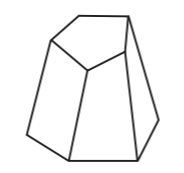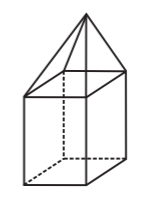Open in App
Not now

# Class 8 NCERT Solutions – Chapter 10 Visualising Solid Shapes – Exercise 10.3

• Last Updated : 24 Nov, 2020

### (i) 3 triangles? (ii) 4 triangles? (iii) a square and four triangles?

Solution:

(i) 3 triangles: No, because polyhedron must have minimum 4 faces i.e all edges should meet at vertices.

(ii) 4 triangles: Yes, as all the edges are meeting at the vertices and has four triangular faces.

(iii) a square and four triangles: Yes, because all the eight edges meet at the vertices having a square face and four triangular faces.

### Question 2: Is it possible to have a polyhedron with any given number of faces? (Hint: Think of a pyramid).

Solution:

Yes, It is possible to have a polyhedron with any given faces only if the number of faces are greater than or equal to four.

### Question 3: Which are prisms among the following?Solution:

Prisms among the given images are

(ii) Unsharpened pencil

(iv) A box.

### Question 4: (i) How are prisms and cylinders alike? (ii) How are pyramids and cones alike?

Solution:

(i) If the number of sides in a prism are increased to certain extent, then the prism will take the shape of cylinder i.e. a prism with a circular base.

(ii) If the number of sides of the pyramid is increased to same extent, then the pyramid becomes a cone i.e. a pyramid with a circular base.

### Question 5: Is a square prism same as a cube? Explain.

Solution:

Yes, a square prism can be same as a cube, but if the height of the prism is greater than It may be cuboid.

### Question 6: Verify Euler’s formula for these solids.

(i)(ii)Solution:

(i) No. of Faces (F) = 7

No. of Vertices (V) = 10

No. of Edges (E) = 15

By Using Euler’s formula: F + V – E = 2 and Substituting the values, we get

⇒ 7 + 10 – 15 = 2

⇒ 2 = 2

Therefore, Euler’s formula is verified.

(ii) No. of Faces (F) = 9

No. of Vertices (V) = 9

No. of Edges (E) = 16

By Using Euler’s formula: F + V – E = 2 and Substituting the values, we get

⇒ 9 + 9 – 16 = 2

⇒ 2 = 2

Therefore, Euler’s formula is verified.

### Question 7: Using Euler’s formula find the unknown.

Solution:

(i)

No. of Faces (F) = F

No. of Vertices (V) = 6

No. of Edges (E) = 12

By Using Euler’s formula: F + V – E = 2 and Substituting the values, we get

⇒ F + 6 – 12 = 2

⇒ F = 2 + 6

⇒ F = 8

Therefore, No. of Faces (F) = 8

(ii)

No. of Faces (F) = 5

No. of Vertices (V) = V

No. of Edges (E) = 9

By Using Euler’s formula: F + V – E = 2 and Substituting the values, we get

⇒ 5 + V – 9 = 2

⇒ E = 2 + 4

⇒ E = 6

Therefore, No. of Vertices (V) = 6

(iii)

No. of Faces (F) = 20

No. of Vertices (V) = 12

No. of Edges (E) = E

By Using Euler’s formula: F + V – E = 2 and Substituting the values, we get

⇒ 20 + 12 – E = 2

⇒ E = 32 – 2

⇒ E = 30

Therefore, No. of Edges (E) = 30

### Question 8: Can a polyhedron have 10 faces, 20 edges and 15 vertices?

Solution:

Since every polyhedron satisfies Euler’s formula, therefore checking if polyhedron can have 10 faces, 20 edges and 15 vertices.

No. of Faces (F) = 10

No. of Vertices (V) = 15

No. of Edges (E) = 20

By Using Euler’s formula: F + V – E = 2 and Substituting the values, we get

⇒ 10 + 15 – 20 = 2

⇒ -5 = 2

As Euler’s formula is not satisfied, therefore polyhedron cannot have 10 faces, 20 edges and 15 vertices.

My Personal Notes arrow_drop_up
Related Articles